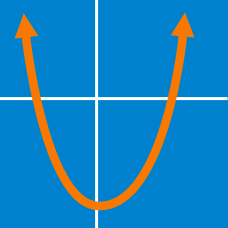Algebra

# Distributive Property - Multiple Terms

If $(x+5)(3x + 8) = Ax^2 + Bx + C$, what is the sum of $A$, $B$, and $C$?

What is the coefficient of $y$ in the expansion of the expression $(4x-y+4)(x+2y-1) ?$

Simplify $\left( 9xy - 6y^2 + 15y \right) \div 3y.$

If $a=-3$ and $b=2$, evaluate $\left(a^2 - 3ab \right) \times \frac{1}{3a} + \left(ab -\frac{b^2}{2} \right) \div 2b.$

Consider the expansion of $(8x+y) (14 y + x)$. After combining like terms, how many terms are there in the expression?

×